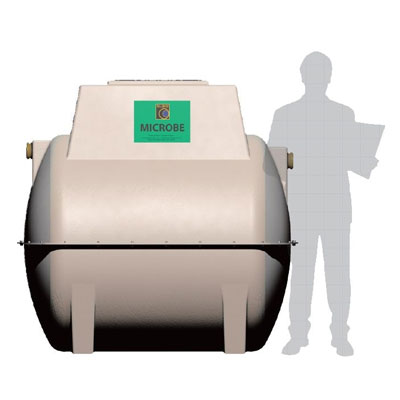Working out what size sewage treatment plant you need can be a lot of hassle. But you can do it easily if you know the details of the project on which you are working. This short guide sets out to answer the question of what size sewage treatment plant you need.

You would probably expect the calculation for this question to simply be based on how many people live in the property. However, you also need to consider that with future use, this number could potentially increase or decrease.

Firstly, sizing a plant takes into account whether the property is a single dwelling or a group of buildings. Secondly, it considers the number of bedrooms in a property.

## Domestic (up to 12 people)

For a single or pair of houses, the calculation is as follows:

##• In the first instance, base your calculation on a lone house with up to three bedrooms on a minimum populace of five people (5P).
• To calculate the size of a treatment system for a single house with more than three bedrooms, add 1P for each extra bedroom. For example, a home with six bedrooms requires a minimum 8P system (5 + 3).
• For a group of two houses with three and four bedrooms, respectively, the system should be a minimum of 11P (5 + 6, as per the guideline above).

## Domestic (12 to 50 people)

When a sewage treatment plant is required for a group of houses with more than twelve occupants, the calculation is a little different. You can make some reductions to allow for the balancing effects on the daily flow of the group of houses, as follows:

• When the total number of people living in the property or properties is 13 to 25 (13 to 25P), multiply it by 0.9 to give an adjusted P-value. For example, for six four-bedroom houses, the total P based on the first part of the calculation would be 36P (6 x 6). The adjusted P would then be 33P (36 x 0.9 = 32.4). Please bear in mind that the P-value should always be rounded up, not down.
• When the total populace is 26 to 50P, multiply it by 0.8. For example, for four three-bedroom and three four-bedroom houses, the total P would be 38P (4 x 5 and 3 x 6). The adjusted P would then be 31P (38 x 0.8 = 30.4).

##